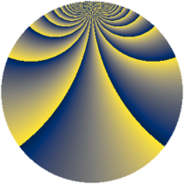# Properties

 Label 3630.2.bnLevel $3630$ Weight $2$ Character orbit 3630.bn Rep. character $\chi_{3630}(41,\cdot)$ Character field $\Q(\zeta_{110})$ Dimension $7040$ Sturm bound $1584$

# Related objects

## Defining parameters

 Level: $$N$$ $$=$$ $$3630 = 2 \cdot 3 \cdot 5 \cdot 11^{2}$$ Weight: $$k$$ $$=$$ $$2$$ Character orbit: $$[\chi]$$ $$=$$ 3630.bn (of order $$110$$ and degree $$40$$) Character conductor: $$\operatorname{cond}(\chi)$$ $$=$$ $$363$$ Character field: $$\Q(\zeta_{110})$$ Sturm bound: $$1584$$

## Dimensions

The following table gives the dimensions of various subspaces of $$M_{2}(3630, [\chi])$$.

Total New Old
Modular forms 32000 7040 24960
Cusp forms 31360 7040 24320
Eisenstein series 640 0 640

## Trace form

 $$7040q + 4q^{3} + 176q^{4} - 10q^{6} - 16q^{9} + O(q^{10})$$ $$7040q + 4q^{3} + 176q^{4} - 10q^{6} - 16q^{9} - 18q^{12} - 56q^{15} + 176q^{16} - 10q^{18} - 60q^{19} + 12q^{22} + 10q^{24} - 176q^{25} + 16q^{27} + 120q^{31} + 6q^{33} + 16q^{34} + 14q^{36} + 24q^{37} + 40q^{39} + 24q^{42} - 20q^{46} + 4q^{48} - 248q^{49} + 12q^{51} + 8q^{55} + 12q^{57} + 72q^{58} + 8q^{60} + 20q^{63} + 176q^{64} + 2q^{66} - 24q^{67} - 44q^{69} - 40q^{72} + 128q^{73} + 6q^{75} - 132q^{76} - 32q^{78} - 68q^{79} + 48q^{81} + 108q^{82} - 20q^{84} + 264q^{85} - 120q^{88} - 88q^{90} + 320q^{91} - 92q^{93} + 80q^{94} + 60q^{97} + 64q^{99} + O(q^{100})$$

## Decomposition of $$S_{2}^{\mathrm{new}}(3630, [\chi])$$ into newform subspaces

The newforms in this space have not yet been added to the LMFDB.

## Decomposition of $$S_{2}^{\mathrm{old}}(3630, [\chi])$$ into lower level spaces

$$S_{2}^{\mathrm{old}}(3630, [\chi]) \cong$$ $$S_{2}^{\mathrm{new}}(363, [\chi])$$$$^{\oplus 4}$$$$\oplus$$$$S_{2}^{\mathrm{new}}(726, [\chi])$$$$^{\oplus 2}$$$$\oplus$$$$S_{2}^{\mathrm{new}}(1815, [\chi])$$$$^{\oplus 2}$$